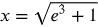1
143
views
50a
Problem

Problem 50a

Chapter 1: Functions and ModelsTextbook ExpertVerified Tutor
19 Oct 2021

Given information

The given equation is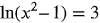Step-by-step explanation

Step 1.

Solve for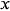given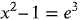use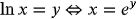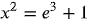add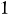on both sides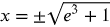take square root on both sides

the natural logarithm is defined only on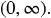So,